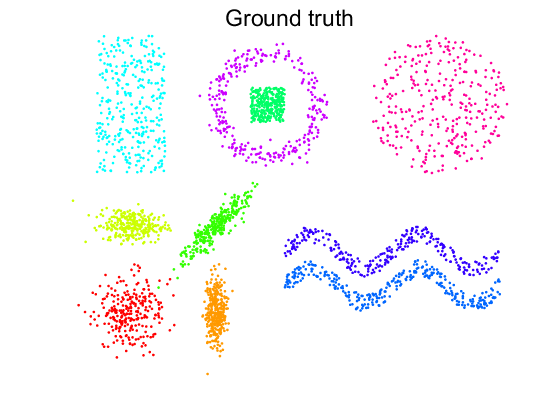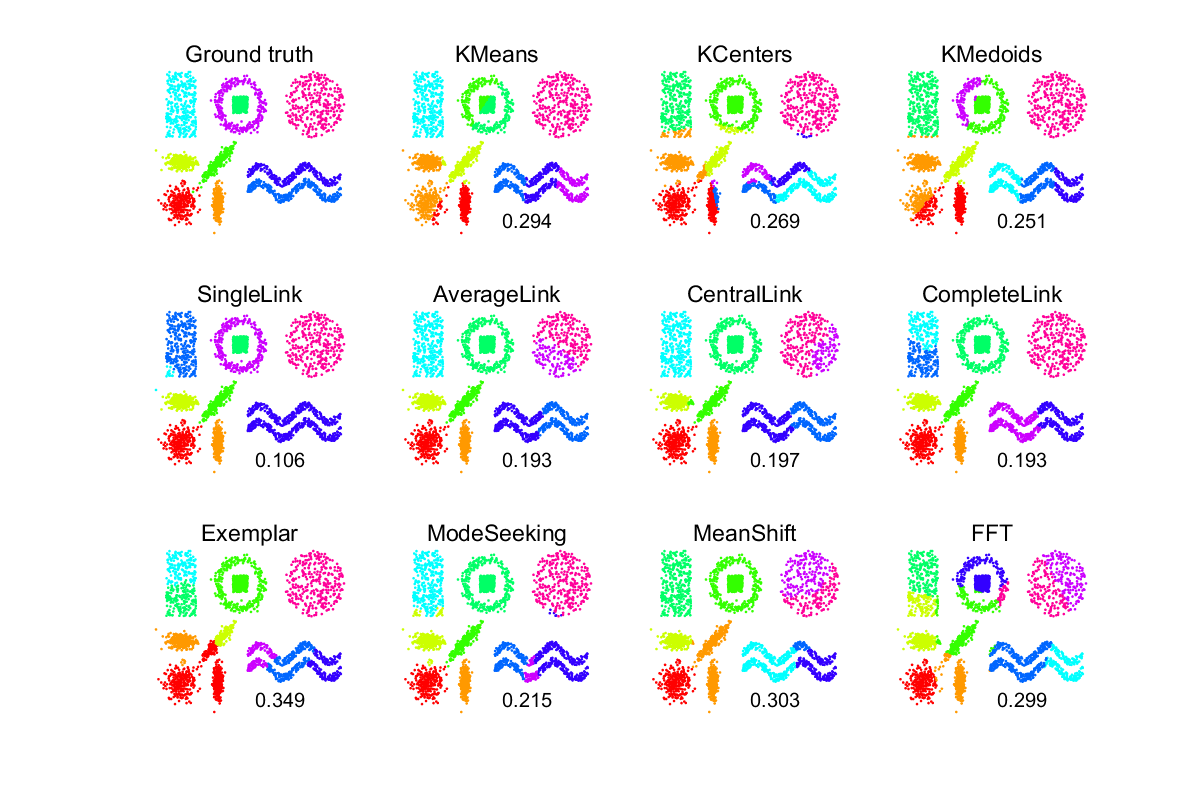# ct_clustprocs Review of cluster procedures.

PRTools and ClusterTools should be in the path

Goto ClusterTools examples for a review of all examples.

It shows the groundtruth of a 2D example with 10 clusters and compares it with the result of 11 cluster procedures. Evaluation is done by assigning all objects in a cluster to the true class of its prototype.

In CLUSTEX1 the same experiment is coded in a more elaborate way.

## Prepare data and set parameters

```randreset   % initialise random generator
k = 10;     % Search for k clusters
n = 1000;   % Speed up hierarchical and exemplar clustering by reducing
% the dataset to at most n points by modeseeking
m = 3000;   % total number of objects in the dataset
x = gendatclust1(m); % generate a 10 cluster problem (3000 objects)
a = +x;     % create unlabeled data
prtime(5)   % stop iterations after some seconds
```

## Show the original data with target classes

```figure;
lab = getnlab(x);
scatn(lab,a,'Ground truth');
```## define cluster routines

The cluster routines are defined as PRTools mappings for creating k clusters. For every cluster procedure its scatterplot for 10 clusters is shown. In addition the classification error is printed based on the assignment of all objects in a cluster to the true class of its prototype.

```proc = cell(1,11);
proc{1}  = clustk(k,'KMeans');             % define KMeans
proc{2}  = clustk(k,'KCenters');           % define KCenters
proc{3}  = clustk(k,'KMedoids');           % define KMedoids
proc{8}  = cluste(k,n);                    % define Exemplar
proc{9}  = clustm(k);                      % define KNN ModeSeek
proc{10} = clusts(k);                      % define Mean Shift
proc{11} = clustf(k);                      % define FFT

figure;
set(gcf,'Position',[100 100 1200 800])
subplot(3,4,1)
lab = getnlab(x);
scatn(lab,a,'Ground truth');
for j=1:11
subplot(3,4,j+1);
scatn(ones(size(x,1),1),x,getname(proc{j}));
text(8,-2,'... in progress ...','FontSize',15);
end

% compute results
for j=1:11
subplot(3,4,j+1);
lab = a*proc{j};
scatn(lab,a,getname(proc{j}));
% compute active learning error based on prototypes
e = clusteval(lab,x,'actl');
text(10,-2,num2str(e,'%5.3f'),'FontSize',15);
drawnow;
end
```
```PR_Warning: EXEMPLAR: Examplar clustering updating stopped by PRTIME after 193 iterations
```